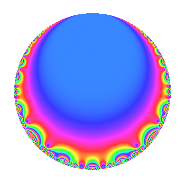Properties

 Label 189.2.a.cLevel 189 Weight 2 Character orbit 189.a Self dual Yes Analytic conductor 1.509 Analytic rank 0 Dimension 1 CM No Inner twists 1

Related objects

Newspace parameters

 Level: $$N$$ = $$189 = 3^{3} \cdot 7$$ Weight: $$k$$ = $$2$$ Character orbit: $$[\chi]$$ = 189.a (trivial)

Newform invariants

 Self dual: Yes Analytic conductor: $$1.5091725982$$ Analytic rank: $$0$$ Dimension: $$1$$ Coefficient field: $$\mathbb{Q}$$ Coefficient ring: $$\mathbb{Z}$$ Coefficient ring index: $$1$$ Fricke sign: $$-1$$ Sato-Tate group: $\mathrm{SU}(2)$

$q$-expansion

 $$f(q)$$ $$=$$ $$q$$ $$\mathstrut -\mathstrut 2q^{4}$$ $$\mathstrut +\mathstrut 3q^{5}$$ $$\mathstrut +\mathstrut q^{7}$$ $$\mathstrut +\mathstrut O(q^{10})$$ $$q$$ $$\mathstrut -\mathstrut 2q^{4}$$ $$\mathstrut +\mathstrut 3q^{5}$$ $$\mathstrut +\mathstrut q^{7}$$ $$\mathstrut +\mathstrut 6q^{11}$$ $$\mathstrut -\mathstrut 4q^{13}$$ $$\mathstrut +\mathstrut 4q^{16}$$ $$\mathstrut +\mathstrut 3q^{17}$$ $$\mathstrut +\mathstrut 2q^{19}$$ $$\mathstrut -\mathstrut 6q^{20}$$ $$\mathstrut -\mathstrut 6q^{23}$$ $$\mathstrut +\mathstrut 4q^{25}$$ $$\mathstrut -\mathstrut 2q^{28}$$ $$\mathstrut -\mathstrut 6q^{29}$$ $$\mathstrut -\mathstrut 4q^{31}$$ $$\mathstrut +\mathstrut 3q^{35}$$ $$\mathstrut -\mathstrut 7q^{37}$$ $$\mathstrut -\mathstrut 3q^{41}$$ $$\mathstrut -\mathstrut q^{43}$$ $$\mathstrut -\mathstrut 12q^{44}$$ $$\mathstrut +\mathstrut 9q^{47}$$ $$\mathstrut +\mathstrut q^{49}$$ $$\mathstrut +\mathstrut 8q^{52}$$ $$\mathstrut -\mathstrut 6q^{53}$$ $$\mathstrut +\mathstrut 18q^{55}$$ $$\mathstrut +\mathstrut 9q^{59}$$ $$\mathstrut -\mathstrut 10q^{61}$$ $$\mathstrut -\mathstrut 8q^{64}$$ $$\mathstrut -\mathstrut 12q^{65}$$ $$\mathstrut -\mathstrut 4q^{67}$$ $$\mathstrut -\mathstrut 6q^{68}$$ $$\mathstrut +\mathstrut 2q^{73}$$ $$\mathstrut -\mathstrut 4q^{76}$$ $$\mathstrut +\mathstrut 6q^{77}$$ $$\mathstrut -\mathstrut q^{79}$$ $$\mathstrut +\mathstrut 12q^{80}$$ $$\mathstrut +\mathstrut 3q^{83}$$ $$\mathstrut +\mathstrut 9q^{85}$$ $$\mathstrut +\mathstrut 6q^{89}$$ $$\mathstrut -\mathstrut 4q^{91}$$ $$\mathstrut +\mathstrut 12q^{92}$$ $$\mathstrut +\mathstrut 6q^{95}$$ $$\mathstrut -\mathstrut 10q^{97}$$ $$\mathstrut +\mathstrut O(q^{100})$$

Embeddings

For each embedding $$\iota_m$$ of the coefficient field, the values $$\iota_m(a_n)$$ are shown below.

For more information on an embedded modular form you can click on its label.

Label $$\iota_m(\nu)$$ $$a_{2}$$ $$a_{3}$$ $$a_{4}$$ $$a_{5}$$ $$a_{6}$$ $$a_{7}$$ $$a_{8}$$ $$a_{9}$$ $$a_{10}$$
1.1
 0
0 0 −2.00000 3.00000 0 1.00000 0 0 0
 $$n$$: e.g. 2-40 or 990-1000 Significant digits: Format: Complex embeddings Normalized embeddings Satake parameters Satake angles

Inner twists

This newform does not admit any (nontrivial) inner twists.

Atkin-Lehner signs

$$p$$ Sign
$$3$$ $$1$$
$$7$$ $$-1$$

Hecke kernels

This newform can be constructed as the intersection of the kernels of the following linear operators acting on $$S_{2}^{\mathrm{new}}(\Gamma_0(189))$$:

 $$T_{2}$$ $$T_{5}$$ $$\mathstrut -\mathstrut 3$$